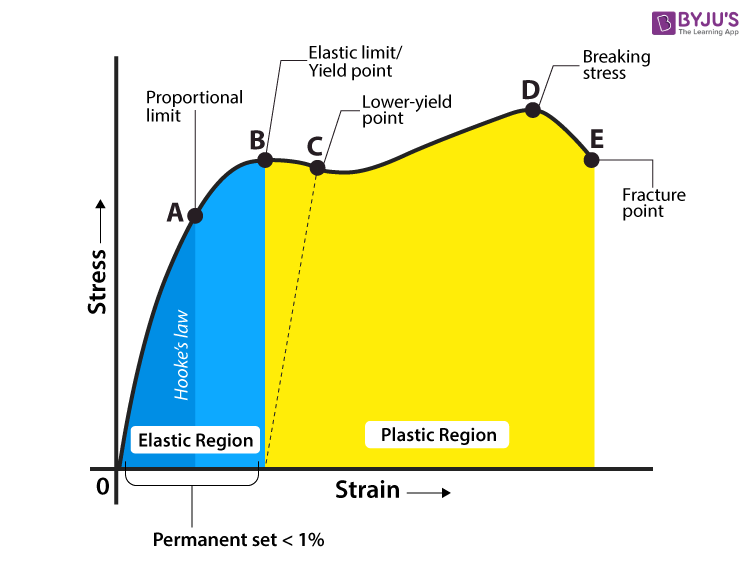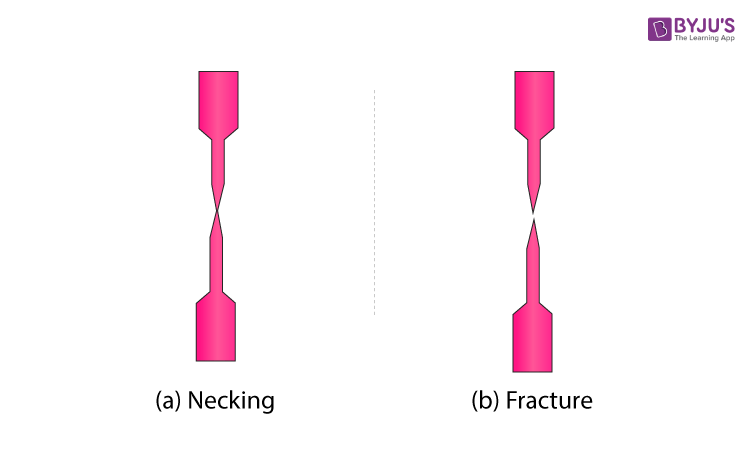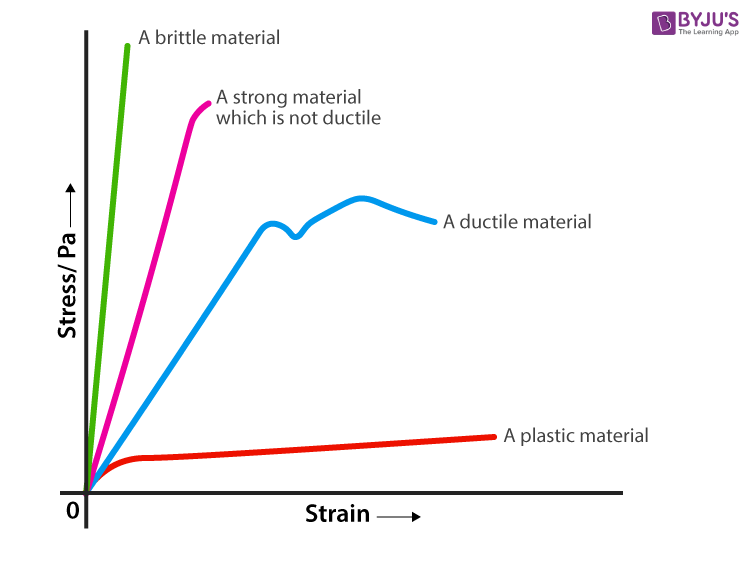Checkout JEE MAINS 2022 Question Paper Analysis : Checkout JEE MAINS 2022 Question Paper Analysis :

# Yield Strength

## What is Yield Strength?

Whether an object is stubborn or malleable is decided by the yield strength. It is the point at which an object ceases to be elastic and becomes plastic. Yield strength helps us choose appropriate materials for the construction based on the requirement. The toys that we so adore were built from something as pliant as plastic and not from metals because it would have been impossible to mould them into the unconventional shapes that we so dearly love. In this article, we shall discuss yield strength in detail.

### Stress-Strain Graph

The strength of a material can be determined by a test known as the tensile test. In this test, the material is mercilessly pulled from both its ends. The relationship between the stress to which the object is subjected to and consequently the strain it suffers can be graphed and this graph is known as the stress-strain graph.From the stress-strain graph given above, we notice that the material initially when stretched behaves like an elastic. Under the elastic limit, the strain caused by the stress is reversible. The material stretches, but once the stress is released, it retains its original length.

Excess stress will deform a material permanently and the application of greater stress results in the formation of what is called a ‘neck’ along with the deformation. Even greater stress will break the neck. The material eventually ceases to the stress and suffers a tragic fracture.### Explanation of Stress-Strain Graph

The stress-strain graph has different points or regions as follows:

• Proportional limit
•  Elastic limit
• Yield point
• Ultimate stress point
• Fracture or breaking point

(i) Proportional Limit

It is the region in the stress-strain curve that obeys the Hooke’s Law. In this limit, the ratio of stress with strain gives us proportionality constant known as young’s modulus. The point OA in the graph is called the proportional limit.

(ii) Elastic Limit

It is the point in the graph up to which the material returns to its original position when the load acting on it is completely removed. Beyond this limit, the material doesn’t return to its original position and a plastic deformation starts to appear in it.

(iii) Yield Point

The yield point is defined as the point at which the material starts to deform plastically. After the yield point is passed, permanent plastic deformation occurs. There are two yield points (i) upper yield point (ii) lower yield point.

(iv) Ultimate Stress Point:

It is a point that represents the maximum stress that a material can endure before failure. Beyond this point, failure occurs.

(v) Fracture or Breaking Point:

It is the point in the stress-strain curve at which the failure of the material takes place.

### Yield Strength Graph

Each and every material possess characteristic stress-strain curve that allows us to determine what application they are best suited for. Each materials curve possesses different points of transition i.e. from elasticity to plasticity and finally to breakage.

• The point at which the material transforms from elastic to plastic is known as the yield point.
• The magnitude of the stress at which the transition from elastic to plastic occurs is known as the yield strength.
• Yield strength is a constant that represents the maximum limit of elastic behaviour.
• Ductile materials like metals have higher yield strength values than plastics.

The stress-strain graph of different materials are given belowThe yield strength of steel and various metals are given in the table below.

 Material Yield Strength (megapascal MPa) Steel 448 Stainless Steel 520 Copper 70 Brass 200+ Aluminium Alloy 414 Cast Iron 130

The yield strength of materials can be increased by adding impurities to the material. The intensified density causes the material to grow more tolerant to deformations, as the impurities fill the voids left after crystalline dislocations.

Stay tuned to BYJU’S to learn more about yield strength formula, deformations and its types with the help of interactive video lessons.

### What is the SI unit of yield strength?

As yield strength is related to deformation which is a result of applied stress, the SI unit of yield strength is N.m-2. In CGS system, the yield strength is g.cm-2.

### State if the given statement is true or false: In drawing deep operations of sheet steels, problems are created by yield point phenomenon.

The given statement is true, yield point phenomenon creates problems during deep drawings. Depressions are created on the sheets during deep drawing and these depressions are undesirable.

### Name one factor which deteriorates the yield strength.

Annealing is one factor which deteriorates the yield strength. Annealing is defined as the process in which heating is done above recrystallization temperature. During the process, the number of dislocations are decreased which results in decrease in the yield strength. The yield strength of a material can be increased by work hardening, grain refinement, and by cold working.

### Give an example of a material which shows yield point phenomenon.

Steel is an example of a material which shows yield point phenomenon. The presence of interstitial atoms are responsible for the yield point phenomenon. For plastic deformation to take place, addition stress needs to be applied so that the atoms will segregate evenly around the dislocations.

### State if the given statement is true or false: Work hardening causes a decrease in yield strength of the material.

The given statement is false because work hardening increases the value of yield strength. Also, it decreases the ductility of the metal.

## The video explains the general stress-strain graph of an elastic material experiencing tensile load.-->
Test Your Knowledge On Yield Strength!